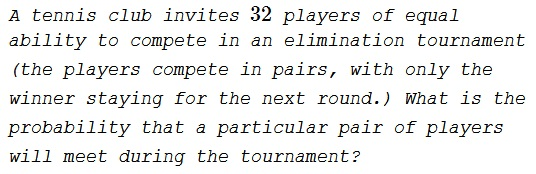# Probability of a Meet in an Elimination Tournament

### Problem### Solution 1

Let's consider a more general problem of $2^k$ players. We shall denote the two players $A$ and $B.$

If $k=1,$ the two players necessarily play each other, $P_1=1.$

If $k=2,$ there are three possible pairings, implying that $A$ and $B$ meet in the first round with the probability of $\displaystyle \frac{1}{3}.$ If not, each wins his first round match with probability $\displaystyle \frac{1}{2},$ such that the probability of their meeting in the second round id $\displaystyle \frac{2}{3}\cdot\left(\frac{1}{2}\right)^2=\frac{1}{6}.$ Thus $\displaystyle P_2=\frac{1}{3}+\frac{1}{6}=\frac{1}{2}.$

In general, with $2^k$ players, $A$ and $B$ meet in the first round with the probability of $\displaystyle \frac{1}{2^k-1}.$ With the probability of $\displaystyle \frac{2^k-2}{2^k-1}$ they do not meet in the first round but continue to the second with the probability of $\displaystyle \left(\frac{1}{2}\right)^2=\frac{1}{4}.$ For the total probability $P_k$ we get a recurrence

$\displaystyle P_k=\frac{1}{2^k-1}+\frac{1}{4}\cdot\frac{2^k-2}{2^k-1}\cdot P_{k-1},$

i.e.,

$\displaystyle 2(2^k-1)P_k=2+(2^{k-1}-1)\cdot P_{k-1}.$

After multiplying both sides by $2^{k-1}$ we get

$2^k(2^k-1)P_k=2^k+2^{k-1}(2^{k-1}-1)\cdot P_{k-1}.$

This recurrence is easily solved:

\displaystyle\begin{align} 2^k(2^k-1)P_k&=2^k+2^{k-1}(2^{k-1}-1)\cdot P_{k-1}\\ &=2^k+2^{k-1}+2^{k-2}(2^{k-2}-1)P_{k-2}\\ &=2^k+2^{k-1}+2^{k-2}+2^{k-3}(2^{k-3}-1)P_{k-3}\\ &\qquad\qquad\cdots\\ &=\sum_{i=k}^{2}2^{i}+2(2-1)P_1=\sum_{i=k}^{1}2^{i}=2\sum_{i=0}^{k-1}2^{i}=2(2^{k}-1). \end{align}

Thus, $2^k(2^k-1)P_k=2(2^k-1),$ so that $P_k=\displaystyle \frac{1}{2^{k-1}}.$

For $k=5,$ the probability is $\displaystyle \frac{1}{16}.$

### Solution 2

With $2^k$ particpants $2^k-1$ games will be played out. On the other hand, the total amount of possible games equal $\displaystyle \frac{2^k(2^k-1)}{2}=2^{k-1}(2^k-1).$ Thus, for each game to be played in the tournament the probability equals $\displaystyle P_k=\frac{2^k-1}{2^{k-1}(2^k-1)}=\frac{1}{2^{k-1}}.$

### Solution 3

Suppose there are $N$ players. When two players meet in round $k$, they are effectively the chosen $2$ from a pool of $2^k$ players who competed in the sub-bracket leading to that particular match. Let us call such a sub-bracket a $k$-sub-bracket.

There are $M_k=2^N/2^k$ $k$-sub-brackets. The probability that both players end up in a particular $k$-sub-bracket is

$\displaystyle P_1(k)=\frac{2^k}{2^N}\cdot \frac{2^k-1}{2^N-1}.$

The probability that two players from a $k$-sub-bracket meet in round $k$ is

$\displaystyle P_2(k)=2\cdot\frac{1}{2^k}\cdot\frac{1}{2^k-1}=\frac{1}{2^{k-1}}\cdot\frac{1}{2^k-1}.$

Thus, the probability that the two players play each other is

\displaystyle \begin{align} P_N&=\sum_{k=1}^{N} M_k\cdot P_1(k)\cdot P_2(k) \\ &=\sum_{k=1}^{N} \left(\frac{2^N}{2^k}\right) \left(\frac{2^k}{2^N}\right) \left(\frac{2^k-1}{2^N-1}\right)\left(\frac{1}{2^{k-1}}\right)\left(\frac{1}{2^k-1}\right) \\ &=\frac{1}{2^N-1}\sum_{k=1}^{N}\frac{1}{2^{k-1}}=\frac{1}{2^{N-1}}. \end{align}

For $N=5$,

$\displaystyle P_5=\frac{1}{16}.$

### Acknowledgment

This is problem 297 Five Hundred Mathematical Challenges by E. J. Barbeau, M. S. Klamkin, W. O. J. Moser (MAA, 1995). Solution 2 was suggested by Xavier Faure; Solution 3 is by Amit Itagi.